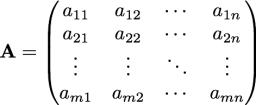# Equations 4648

Solve the system of equations by any method and perform the test:

2 (x + y) -3 (y + 2) = -1
x + 2 / 3y-6 = 2

x =  4.8571
y =  4.7143

### Step-by-step explanation:

2(x+y)-3(y+2)= -1
x+2/3y-6=2

2·(x+y)-3·(y+2)= -1
x+2/3·y-6=2

2x-y = 5
3x+2y = 24

Pivot: Row 1 ↔ Row 2
3x+2y = 24
2x-y = 5

Row 2 - 2/3 · Row 1 → Row 2
3x+2y = 24
-2.33y = -11

y = -11/-2.33333333 = 4.71428571
x = 24-2y/3 = 24-2 · 4.71428571/3 = 4.85714286

x = 34/7 ≈ 4.857143
y = 33/7 ≈ 4.714286

Our linear equations calculator calculates it.Did you find an error or inaccuracy? Feel free to write us. Thank you!

Tips for related online calculators
Do you have a system of equations and looking for calculator system of linear equations?